# 1st PUC Physics Model Question Paper 1 with Answers

Students can Download 1st PUC Physics Model Question Paper 1 with Answers, Karnataka 1st PUC Physics Model Question Papers with Answers helps you to revise the complete Karnataka State Board Syllabus and score more marks in your examinations.

## Karnataka 1st PUC Physics Model Question Paper 1 with Answers

Time: 3.15 Hours
Max Marks: 70

General Instructions:

1. All parts are compulsory.
2. Draw relevant figure / diagram wherever necessary.
3. Numerical problems should be solved with relevant formulae.

Part – A

I. Answer the following questions: ( 10 x 1 = 10 )

Question 1.
Write the dimensional formula for Pressure.
[pressure] = [M1 C-1T-2]

Question 2.
When does the circular motion become uniform?
The circular motion of bodies will be uniform, for uniform speed of particles.

Question 3.
What is the amount of work done by the gravitational force on the body moving on the horizontal plane?
Zero

Question 4.
What is elastic collision?
A collision in which linear momentum and kinetic energy of the interacting system are conserved, is known as an elastic collision.Question 5.
Give one example for elastomers.
Rubber

Question 6.
What is thermal stress?
When thermal expansion is not allowed in a solid, the corresponding stress developed in it is known as thermal stress. The solid acquires a compressive strain due to the external forces, provided by (say) the rigid support at one end of it.

Question 7.
Define mean free path of the molecule in the gas.
The average distance traveled by the molecule during two consecutive collisions is called mean free path.

Question 8.
Where does Kinetic energy of the oscillating particle become maximum?
The K.E of oscillating particle becomes maximum when it reaches the mean position.

Question 9.
What is resonance?
If the frequency of forced oscillation of a system is the same as the natural frequency of the system which induces oscillation in the other, then both the systems are said to be in resonance.Question 10.
Give the value of phase difference between the particles of adjacent loop of stationary wave.
Φ = 180°

Part – B

II. Answer any five of the following questions:  ( 5 x 2 = 10 )

Question 11.
Name any two fundamental forces of nature.

• Gravitational force
• Electro – weak force
• Strong nuclear force

Question 12.
Mention any two sources of systematic error.

• Instrumental error.
• Personal error.

Question 13.
Draw position time graph for the particle having zero acceleration.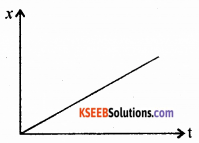Question 14.
Write the equation for maximum horizontal range in projectile motion and explain the terms.
R = $$\frac{u^{2} \sin 2 \theta}{g}$$
Where u – initial speed,
θ – angle of projection
g – acceleration due to gravity
R – Horizontal rangeQuestion 15.
Mention any two methods of reducing friction.

1. Wear and tear can be minimised by polishing the surfaces and lubricating machinery parts with oil and grease etc.
2. Streamlining the bodies reduce fluid (air or water) resistance.

Question 16.
Mention the conditions for the body in mechanical equilibrium.
(1) The vector sum of the forces on the rigid body is zero for translatory equilibrium
$$\sum_{i=1}^{n} \vec{F}_{i}=0$$ and (acceleration a = 0 )
(2) The vector sum of the torques on the rigid body is zero for rotatory equilibrium (a=0) i.e.i.e. the components of X, Y and Z independently vanish to zero for linear equilibrium.Sum of X components, Y components and Z components of torque on the particles, vanish for rotational equilibrium.

Question 17.
State and explain Hooke’s law.
Statement: The ratio of stress to strain is a constant for a material within the elastic limit.
Modulus of elasticity = $$\frac{\text { Stress }}{\text { Strain }}$$

Within the elastic limit, stress v/s strain is a straight line ‘A’ is the elastic limit upto which Hooke’s law is applicable. Beyond ‘B’ the yielding point, the wire extends but does not return to the initial state when the deforming force is removed. ‘F’ is the breaking point. ‘EF’ allows the material to be malleable and ‘DE’, ductile.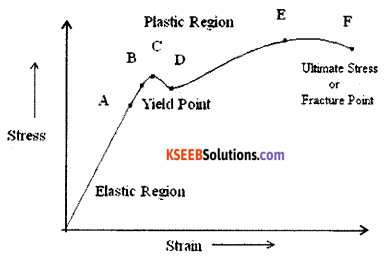Question 18.
What are extensive thermodynamical variables? Give one example.
Internal energy, volume, mass are called extensive thermodynamic variables.

Part – C

III. Answer any FIVE of the following questions : ( 5 x 3 = 15 )

Question 19.
Obtain.the equation for maximum height in projectile motion.
We know that (vocosθ) remains constant.
Instantaneous distance along the horizontal is given by ,
x = (vocosθ)t
for x = R (range of the projectile), t = T = time of flight.Question 20.
State and prove the law of conservation of linear momentum.
Statement: In an isolated system of collision of bodies, the total linear momentum before impact is equal to the total linear momentum after impact.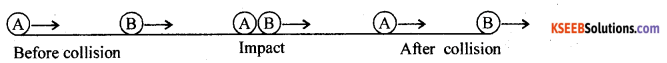Let m1 and m2 be the masses of two bodies moving along $$\vec{v}_{1 i}$$ and $$\vec{v}_{2 i}$$. Let $$\vec{v}_{1 f}$$ and be the $$\vec{v}_{2 f}$$ be final velocities after the impact.
At the time of impact the force of action acts on the body B and the force of reaction acts on A.
Applying Newton’s III law of motion
$$|Force of action on \mathrm{B}|=-| Force of reaction on \mathrm{A} |$$This shows that the total final linear momentum of the isolated system equals its total initial momentum.Question 21.
Prove the work energy theorem in the case of constant force.
w.k.t work done W = $$\overrightarrow{\mathrm{F}} \cdot \overrightarrow{\mathrm{s}}$$
where F = ma, s = $$\frac{v^{2}-u^{2}}{2 a}$$
W = $$\overrightarrow{\mathrm{F}} \cdot \overrightarrow{\mathrm{s}}$$ becomes
W = $$\frac{m\left(v^{2}-u^{2}\right)}{2}$$
or W = Kf – Ki
or W = Δ K.E
Thus, work come by a constant force is equal to the difference in the K.E of the body.

Question 22.
Obtain the equation for moment of couple.
Two equal and opposite forces acting at two different points constitute couple. The moment of couple is measured by taking the product of magnitude of any one force and the arm of the couple.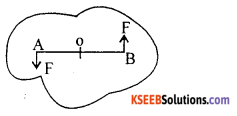Hence moment of couple = $$\overrightarrow{\mathrm{F}}|\overrightarrow{\mathrm{OA}}|+\overrightarrow{\mathrm{F}}|\overrightarrow{\mathrm{OB}}|=\overrightarrow{\mathrm{F}}(\mathrm{OA}+\mathrm{OB})=\overrightarrow{\mathrm{FAB}}$$

Question 23.
Derive equation for acceleration clue to gravity on the surface of the earth.
Let M be the mass of the Earth and R be its radius.
We know that F = $$-\frac{\mathrm{GMm}}{\mathrm{r}^{2}}$$
where ‘m’ is a mass body very close to the surface of the Earth.
Force of gravity on the mass ‘m’ is F’ = mg
However F = F’
Therefore mg = $$\frac{\mathrm{GMm}}{\mathrm{R}^{2}}$$
i.e, g = $$\frac{\mathrm{GM}}{\mathrm{R}^{2}}$$Question 24.
Show that volume co-efficient of expansion of ideal gas is inversely proportional to absolute temperature.
At constant pressure, V ∝ T
i.e., V = KT. For an increase in the temperature ∆T, the corresponding increase in the volume of the gas will be ∆W.
i.e., (V + ∆V) = K(T + ∆T)
i.e., ,V + ∆V = KT+ K∆T
i.e., $$\frac{\Delta \mathrm{V}}{\Delta \mathrm{T}}=\mathrm{K}=\frac{\mathrm{V}}{\mathrm{T}}$$
or
$$\frac{\Delta \mathrm{V}}{\mathrm{V} \Delta \mathrm{T}}=\frac{1}{\mathrm{T}}=\gamma$$
where r is volume co-efficient of an ideal gas

Question 25.
Show that Cv = $$\frac{3}{2} \mathbf{R}$$ for mono atomic gas.
(dQ) = du = Cv dT
ie. Cv dT = $$\frac{f \mathrm{R} d \mathrm{T}}{2}$$
∴ Cv = $$\frac{f R}{2}$$ for mono atonic gas, f = 3
Cv = $$\frac{3 \mathrm{R}}{2}$$

Question 26.
Give any three differences between progressive wave and stationary wave.

• Progressive waves transfer energy, whereas stationary waves do not.
• No particles of the medium are in a state of rest in a PW but in a SW, the particles at nodes will be at rest.
• The particle velocities will remain the same for a given displacement in a PW, whereas in a SW, particles at nodes will have zero speed and at anti-nodes, particles have maximum speed.
• y = f(x, t) for a PW and y = f(x) g(t) for a SW.
• Distance between two points at which particles have same state of vibration is called , wavelength in a PW whereas distance between two consecutive nodes or anti-nodes is half the wavelength.

Part – D

IV. Answer any TWO of the following questions : ( 2 x 5 = 10 )

Question 27.
Derive equation for centripetal acceleration.
Let $$\vec{r}$$ and $$\vec{r}^{\prime}$$ be the position vectors and $$\vec{v}$$ and $$\vec{v}^{\prime}$$ veIocities of the object when it is at point P and P’. By definition, velocity at a point is along the tangent at that point in the direction of the motion. Since the path is circular $$\vec{v}$$ is perpendicular to $$\vec{r}$$ and $$\vec{v}^{\prime}$$ is perpendicular to $$\vec{r}^{\prime}$$.
Therefore, ∆$$\vec{v}$$is perpendicular to ∆$$\vec{r}$$ Average acceleration $$\frac{\Delta \vec{v}}{\Delta t}$$ is perpendicular to ∆$$\vec{r}$$.
The magnitude of $$\vec{a}$$ is, by definition, given by $$|\vec{a}|=\lim _{\Delta t \rightarrow 0} \frac{|\Delta \vec{v}|}{\Delta t}$$
The triangle formed by the position vectors is similary to the triangle formed by the velocity vectors.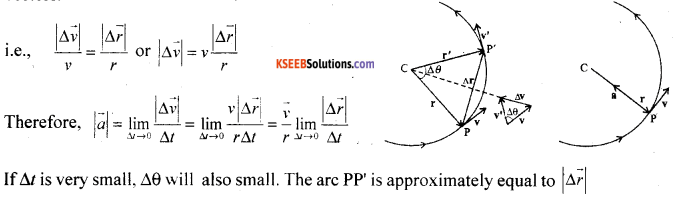lf ∆t is very small. ∆ will also small. The arc PP is approximately equal to $$|\Delta \vec{r}|$$
i.e., $$\lim _{\Delta t \rightarrow 0} \frac{|\Delta \vec{r}|}{\Delta t}$$ = v. Thus, centripetal acceleration $$|\vec{a}|=\frac{v}{r} v=\frac{v^{2}}{r}$$ and $$\vec{a}=\frac{v}{r} \frac{d \vec{r}}{d t}$$.
The centripetal acceleration is always directed towards the centre. The centripetal force = ma.Question 28.
Energy can neither be created nor destroyed but can be transformed into other forms of energy.
Let a particle be at ‘C’. Initially the particle w ill be zero velocity. Let ‘h’ be the height of the particle.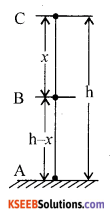P.Ec = mgh, K.Ec = 0
T.E = mgh
At B, K.E = $$1 / 2 \mathrm{mv}_{\mathrm{B}}=1 / 2 \mathrm{m}(2 \mathrm{g} x)=\mathrm{mg} x$$
P.EB = mg (h – x)
Hence T.E = (mgh – mgx) + (mgx)
= mgh
At A, vA2= 2gh
So that K.E = $$\frac{1}{2}$$ m2gh = mgh
P.EA = O.
Hence at all points A, B and C, the total energy is conserved.

Question 29.
Obtain equation for Kinetic energy of rolling motion.
K.E of rolling body = Transiatory K.E + Rotational K.EV. Answer any TWO of the following questions : ( 2 x 5 = 10 )

Question 30.
State and prove Bernaulli’s theorem.
Along a steam line, in a steady flow of non viscous fluid, potential energy, kinetic energy and pressure energy remain constant.
i.e., $$\mathrm{mgh}+1 / 2 \mathrm{mv}^{2}+\mathrm{PV}=\mathrm{K}$$
i.e., $$\mathrm{mgh}+1 / 2 \mathrm{mv}^{2}+\mathrm{Pm} / \rho=\mathrm{K} \div \mathrm{m}$$
we get, $$g h+\frac{v^{2}}{2}+\frac{P}{\rho}=K \div g$$
i.e., $$\mathrm{h}+\frac{v^{2}}{2 g}+\frac{\mathrm{P}}{\rho g}=\mathrm{K}$$
where h is called gravitational head, $$\frac{v^{2}}{2 g}$$ is velocity head and $$\frac{P}{\rho g}$$ is pressure head.
For a horizontal Flow, $$\frac{\rho v^{2}}{2}+\frac{P}{\rho g}$$ = K
As the velocity of the fluid increases, pressure of the fluid decreases.

Question 31.
What is isothermal process? Obtain equation for work done in isothermal process.
The process in which changes in pressure and volume of a gas system takes place at constant temperature of the system, is known as isothermal process.
For an isothermal process, PV = constant.
At any intermediate stage with pressure P and change in volume from V to V + dV,
work done dw = P dV. However for the entire process W = $$\int_{V_{1}}^{V_{2}} P d V$$ .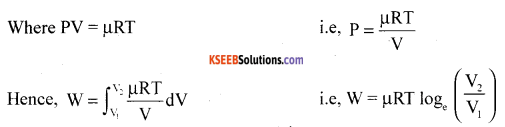For V2 > V1, W > 0 and for V2 < V1, W < 0.
In an isothermal expansion, the gas absorbs heat and does work, while in an isothermal compression, work is done on the gas by the environment and heat is released.Question 32.
What is closed pipe? Show that modes of vibration in closed pipe are odd harmonics.
Let ‘L’ be the length of the closed pipe. A pipe with one end closed is known as a closed pipe system. Le V be the velocity of sound in air. Length of half segment = $$\frac{\lambda_{0}}{4}$$
i.e., L = $$\frac{\lambda_{0}}{4}$$ i.e., λ0 = 4L.The least mode of vibration is called fundamental mode i.e.,
fundamental frequency f0 = $$\frac{\mathbf{v}}{\lambda}=\frac{\mathbf{v}}{4 L}$$
In the second mode of vibration.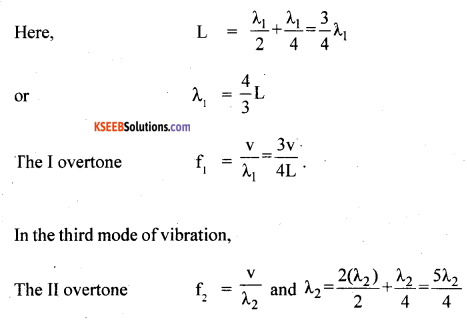i.e., f2 = $$\frac{5 v}{4 L}$$
Hence f0 : f1 : f2 :……..: fn : : 1 : 3 : 5 :………: (2n – 1)

VI. Answer any THREE of the following questions : ( 3 x 5 = 15 )

Question 33.
A car moving along a straight road with speed of 144 Kmh is brought to a stop within a distance of 200 m. What is the retardation of the car and how long does it take to come to rest?
Given u = 144 kmh-1
ie., u = $$\frac{144 \times 5}{18} \mathrm{ms}^{-1}$$
ie., u = 40 ms-1
v = 0, s = 200 m, a = ?   t = ?
w.k.t v2 = u2 + 2aS
i.e., 0 = (40)2 + 2a(200)
a = $$\frac{-1600}{400}$$
a = -4ms-2
By using, v = u + at,
0 = 40 – 4t
i.e, t = $$\frac{40}{4}$$ =10 s
Hence time taken by the car to stop
t = 10sQuestion 34.
A circular racetrack of radius 200 m is banked at the angle of 10°. If the coefficient of friction between the wheels of racecar and the road is 0.15 what is the
(a) optimum speed of the race car to avoid wear and tear on its tyres?
(b) maximum permissible speed to avoid slipping? [Given: Acceleration due to gravity on the earth = 9.8 ms-2]
(i) Give r = 200 m, θ = 10°, μ = 0.15
v = vmax = ?   g = 9.8 ms-1
w.k.t  vmax = $$\sqrt{\frac{r g(\mu+\tan \theta)}{(1-\mu \tan \theta)}}$$Question 35.
If the weight of a 4 kg mass on the surface of the earth is 39.2 N, calculate the acceleration due to gravity of earth at
(a) 32 km height from the surface of the earth.
(b) 16 km depth from the surface of the earth. [Given : Radius of earth = 6400 km]
Given m = 4kg, F = W = 39.2 N
(i) with attitude
$$g^{\prime}=g\left(1+\frac{h}{R}\right)^{-2}$$
given g = 10ms-1, h = 32 km, h <<R
hence g1 = g(1 – 2h/R)
i.e., g1 = 9.8 $$\left(1-\frac{2 \times 32}{6400}\right)$$ = 9.8 x 0.99
g1 =9.702 ms-2
(ii) with depth; g1 = g( 1 – h/R)
i.e., g1 = 9.8 $$\left(1-\frac{16}{6400}\right)$$
i.e., g1 = 9.8 x 0.9975
g1 = 9.775 ms-2Question 36.
A copper block of mass 2.5 kg is heated in a furnace to a temperature of 500°C and then placed on a large ice block. What is the maximum amount of ice that can melt?
Given m = 2.5 kg θ1 = 500°C, θ2 = 0°C
Cs =0.39 x 103 Jkg-1k-1
L = 3.35 x 105 Jkg-1,  μice = ?
Since mice = Cs Δ θ = miL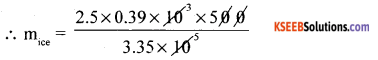i.e., mice = 1.455 kgQuestion 37.
A body oscillates with SHM according to the equation (in SI units)
x = 5 cos $$\left(3 \pi+\frac{\pi}{4}\right)$$
Calculate : (a) frequency of oscillation (b) amplitude of oscillation (c) Displacement of oscillation at t = 1s
given,  x = 5 cos (3πt + π/4)m,
f = ?   A = ?   x = ?   when t = 1s
Comparing this with x = A cos (wt + Φ) we get,
(i) A = 5m
i.e, x= $$-5 / \sqrt{2}$$ = -3.535 m